### Skip List Data Structure

Skip list is an efficient data structure to store sorted elements. Skip list augments the normal linked list with more metadata to provide better time complexity. Like balanced trees, skip list provides O(logN) time complexity for search/insert/delete. In this post, we review the skip list data structure and see how we can optimize its operation with finger search. We present a simple C++ implementation and provide some experimental results with this implementation. We compare skip list with balanced search trees, and see how for concurrent programs skip list is the clear winner.

## Structure

A skip list can be used as an ordered set/map. Internally, a skip list is a linked list with more metadata. Each element is represented by a node in the linked list. Unlike a normal linked list where each node has exactly one forward pointer to the next element in the list, in a skip list, a node can have more than one forward pointer. We define the level/height of a node as the number of its forward pointers.

Forward pointers property: The ith forward pointer of a node points to the next node of level i or higher. We denote the ith forward pointer of node x with x→forward[i]

When we insert an element into a skip list, we generate a node with a random level in [1, MaxLevel], and we set its forward pointers such that we keep the forward pointers property stated above. A skip list has a parameter p that specifies the fraction of nodes in each level that also show up in the next higher level. For example, with p=0.5, half of the nodes in level 1 also show up in level 2. Similarly, 0.25 of all nodes also show up in level 3, and so on. The header of a skip list is a dummy node at the beginning of the list that has a forward pointer to the first nodes in all levels. We define the level of a skip list as the level of the header node. At the end of the list, there is another dummy node called NIL with a key larger than any possible key. The last node of all levels points to NIL. At initialization, the level of the list is 1, and all forward pointers of the list's header point to NIL

## Basic Operations

### Search

We start with the header node and search level-by-level until we reach level 1. In each level, we search for node x that its key is smaller than and the key of the node after that is larger than or equal to the key that we are looking for. When we find x, we go to the next level and repeat the above process. When we are done with the search in level 1, we have reached the position that the key must exist. Specifically, now x is exactly one node before the target node. So, we move one step by x = x→foraward. Now, if x→key is our desired key, we return the value of x. Otherwise, we return failure meaning an element with the given key does not exist in the skip list.Figure 2. An example of a search in a skip list. We are looking for the element with key equals to 31. The skip list allows us to skip some elements that we would have to visit if we used a normal linked list.Do we have to start to search at the top level?
No. You can start searching at any level you like. If the item exists, you will find it no matter at which level you start searching. If you search at level 1, you are basically searching linearly like a normal linked list. Thus, you will get O(n). By start searching from a higher level, we may benefit from passing some items quickly, but on the other hand, we may do some basically useless comparisons if we start at a very large level. Thus, there is a trade-off. We will see that there is a sweet spot that results in the best average performance. We typically set the MaxLevel of the skip list to this value and always start searching from the top level.

### Insert

For the insert, we basically search for the key with the same procedure as explained above. The only difference is when we are doing the level-by-level search, we keep track of the nodes in each level that are right before given key. These nodes must update their forward pointers. We call them to_be_updated nodes. If a node with the given key already exists, it is simple; we just update its value. Otherwise, we create a new node and add it to the structure by updating the forward pointers of the to_be_updated nodes. We assign a random level for the new node. If the new level is larger than the current level of the skip list, we set to_be_updated[i] for all i larger than the current level to the header. Finally, we update the forward pointers of the to_be_updated nodes and the newly added node simply just like the normal linked list for each level.

`Insert(list, searchKey, newValue) to_be_updated[1..MaxLevel] x := list→header for i := list→level downto 1 do while x→forward[i]→key < searchKey do x := x→forward[i] to_be_updated[i] := x x := x→forward if x→key = searchKey then x→value := newValue else newLevel := randomLevel() if newLevel > list→level then         for i := list→level + 1 to newLevel do     to_be_updated[i] := list→header         list→level := newLevel x := makeNode(newLevel , searchKey, value) for i := 1 to newLevel do x→forward[i] := to_be_updated[i]→forward[i] to_be_updated[i]→forward[i] := x`
Figure 3. Skip list insert algorithm

We generate the random level for the new node as follows:

`randomLevel() lvl := 1 while random() < p and lvl < MaxLevel do lvl := lvl + 1 return lvl`
Figure 4. Random level generation algorithm

### Delete

Delete is very similar to insert, except for delete, we only update items from to_be_updated that actually points to the node to be deleted. After deleting the node, we might need to change the value of the current level of the skip list, as the deleted node might happen to be the node with the largest level, and now that we deleted it we have to change the level. Thus, we check the header; while the forward pointer of the current level is pointing to NIL, we decrease the level of the skip list.

`Delete(list, searchKey) to_be_update[1..MaxLevel] x := list→headerfor i := list→level downto 1 do while x→forward[i]→key < searchKey do x := x→forward[i] to_be_update[i] := x x := x→forward if x→key = searchKey then for i := 1 to list→level do if to_be_update[i]→forward[i] ≠ x then break to_be_update[i]→forward[i] := x→forward[i] free(x) while list→level > 1 and list→header→forward[list→level] = NIL do list→level := list→level – 1`
Figure 5. Skip list delete algorithm

### Duplicate Elements

To support having elements with duplicate keys, we have to change the algorithm such that we always do the insert instead of updating the value of the existing element. We do not need to change search and deletion. This way, the search returns the most recently added element with the given key, and delete also removes the most recently added element.

### How to set MaxLevel?

The best level to start the search is level L with 1/p nodes . For example, if p = 0.5, the best level to start is the level that we expect to have 1/0.5 = 2 nodes. The value L depends on how many items we have in our skip list. Specifically, L(n) = log1/pn. Since this is the best level to start, we set MaxLevel to  L(N) where N is the maximum number of elements that we expect to put in the skip list.

## Time and Space Complexity

The time complexity of the search, insert, and delete are the same and all depends on how long it takes to find the element with the given key.  provides an analysis that shows the expected value of the upper bound cost of finding an element is L(n)/p + 1/(1-p) + 1 with variance (L(n) – 1)(1–p)/p2 + (1 – 1/np)/p + p/(1–p)2. Since L(n) =log1/pn, we see that all skip list operations are of O(log1/pn).  The average number of pointers per node is 1/(1-p). Thus, the average number of pointers is n/(1-p) which results in O(n) space complexity. Note that we set the MaxLevel to Log1/pN which is undefined for p equal to 0 or 1. Thus, 0 < p < 1
Time complexity: O(log1/pn)
Space complexity: O(n)

## Optimization: Locality of Reference and Search Fingers

For some applications, we might have this assumption that the keys that we are looking for are close to each other most of the time. This locality of reference provides an opportunity to improve the search because we know the area of the linked list that we need to search for the key. This optimization technique is called finger searching and can be used with other search structures as well. For a skip list, a good finger is the array of the rightmost elements in each level left to the last element that we searched. This finger array is the to_be_updated array in the insert and delete operations.

To search the element with the given key, we first compare it with the finger. If the searchKey is on the right side of finger→key, we start from level 2 and find the largest level lvl such that finger[lvl]→forward[lvl] < searchKey. Then, we start our search finger[lvl]. The reason for looking for the largest lvl in this case, is that by starting from a higher level we may skip more elements. But on the other hand, we don't want to start from a level that is too high such that the next element in that level skip the searchKey itself, as such skip is not useful. In other words, we like to skip, but only if the skip keeps us before the searchKey, that's why we increase the lvl as long as the finger[lvl]→forward[lvl] < searchKey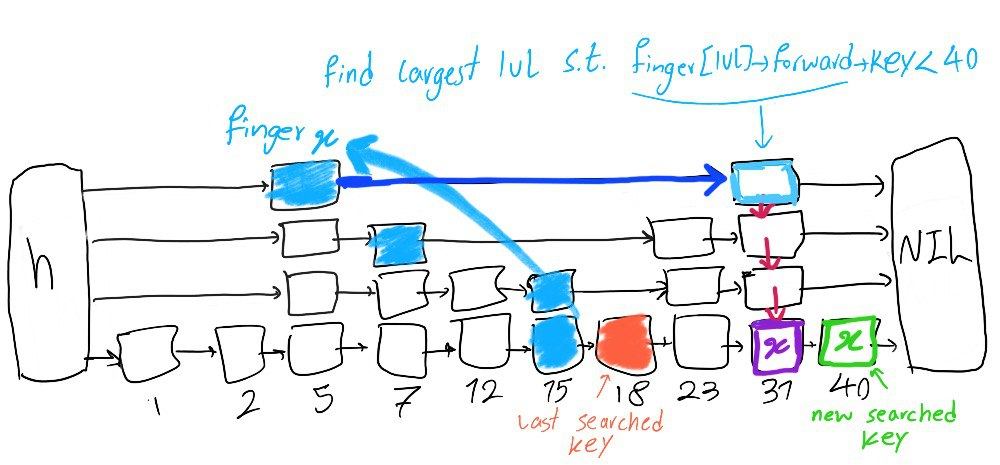Figure 6. Finger search when new searched key is on the right side of the finger (forward search)
If the searchKey is not on the right side of the finger→key, we find the smallest level lvl such that finger[lvl]→key < searchKey. We may not find such lvl. In this case, we start from the header and the top level like the normal search without fingers. If that happens, our search to find the first finger[lvl] with key smaller than searchKey is wasted. Thus, when we have poor locality of reference, finger searching results in a slower search.Figure 7. Finger search with the new searched key is on the left side of finger (backward search)`SearchWithFinger(list, searchKey)lvl := 2if list→finger→key < searchKey then-- move forward, find the largest lvl s.t. list→finger[lvl]→forward[lvl]→key < searchKeywhile lvl ≤ list→level and list→finger[lvl]→forward[lvl]→key < searchKey do lvl := lvl + 1lvl := lvl – 1x := list→finger[lvl]else-- move backward, find the smallest lvl s.t. list→finger[lvl]→key < searchKeywhile lvl ≤ list→level and list→finger[lvl]→key ≥ searchKey do lvl := lvl + 1if lvl > list→level thenlvl := list→levelx := list→headerelse x := list→finger[lvl]for i := lvl downto 1 dowhile x→forward[i]→key < searchKey do x := x→forward[i]list→finger[i] := xx := x→forwardif x→key = searchKey then return x→valueelse return failure`Figure 8. Skip list finger search algorithmWhy in the first case, we look for the largest lvl, and in the second case we search for the smallest lvl?
Whether we start from higher levels or lower levels depends on the location of the level 1 finger. If we are on the left side of the key, we like to go as high as we can to get useful skips. If we are on the right side of the key, we want to find the first finger that is before the key. Thus, it depends on our searching direction, to search forward, we prefer higher levels, to search backward, we prefer the first level that is before the key.

### Time Complexity with Fingers

  proves that the average number of comparisons to find an element k step away from the finger is L(k) + L(k)/p + 2p/(1–p) + 4. Thus, the finger search is of order O(log1/pk) which is a big deal for a skip list with a large number of elements when we have locality of reference, i.e., k is small.

## Experimental Results

In this section, we want to see how a simple C++ implementation of skip list works in practice. You can obtain the code used for these experiments from . For each experiment, we first load the data in order, and then do the search and erase on random keys. The benchmarking code is also provided in .

### The Effect of Number of Elements

First, let's examine the time complexity in practice by changing the number of elements in the skip list. Figure 9 shows the effect of the number of elements on the average search time. Unless stated otherwise, for all experiment we set p = 0.25 and MaxLevel = L(n). Based on theoretical results, we know skip list search is of O(log1/pn).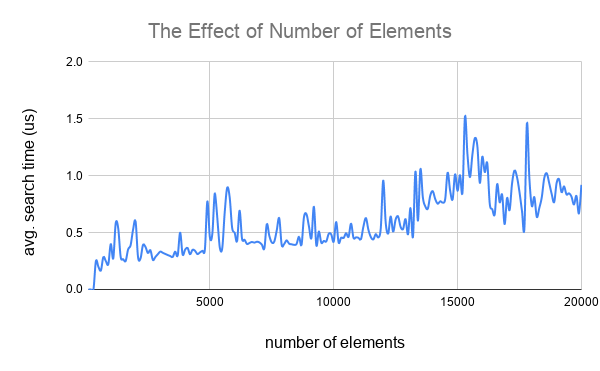Figure 9. The effect of the number of elements on the average search time

Figure 10 compares the average search time of skip list with that of the normal linked list (i.e., a skip list with MaxLevel = 1). The difference between O(n) time complexity of the linked list and O(log1/pn) time complexity of the skip list is clear.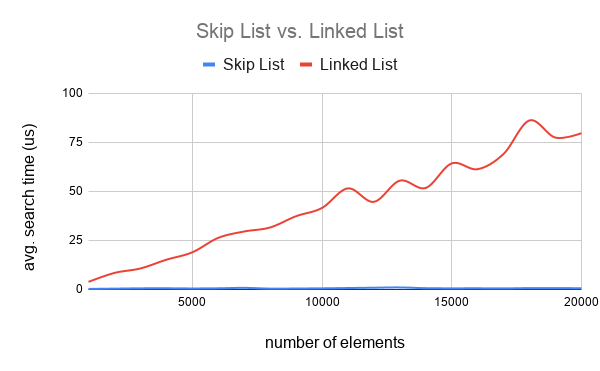Figure 10. Skip list vs. linked list regarding the average search time

Now, let’s examine the space complexity. For the space complexity, we count the number of pointers. From theoretical results, we know the average number of pointers for a skip list with n elements is n/(1-p). Figure 11, shows the experimental number of pointers and compares it with n/(1-p) which confirm the theoretical results.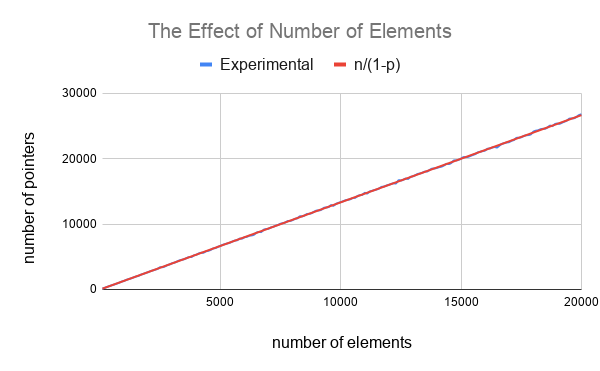Figure 11. The effect of the number of elements on the number of pointers

The time efficiency of the skip list comes with an additional space cost.  Figure 12 shows how the number of pointers of a skip list changes compared with that of a linked list.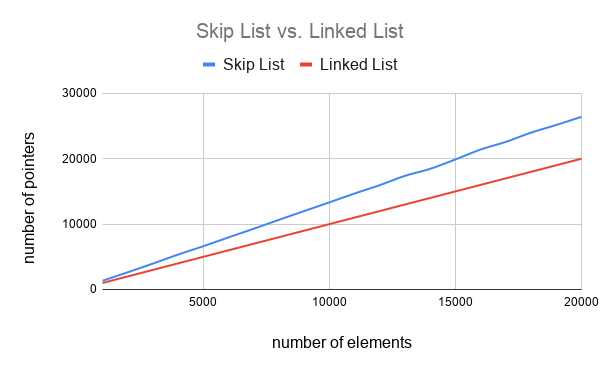Figure 12. Skip list vs. linked list regarding the number of pointers

### The Effect of MaxLevel

Figure 13 shows the effect of MaxLevel on the average search time on a skip list with 100000 elements and p = 0.25. As expected by increasing MaxLevel the search time decreases. Note that the suggested MaxLevel is L(100000) = Log4100000 = 8.3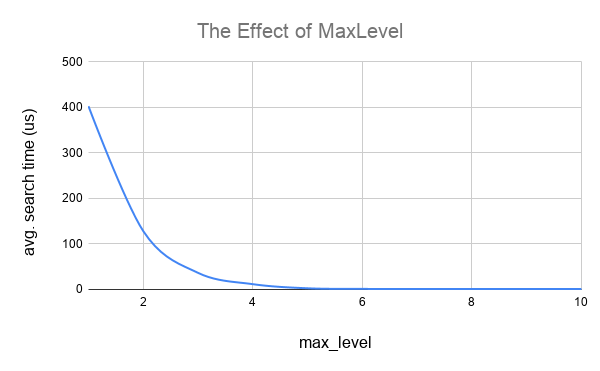Figure 13. The effect of MaxLevel on the average search time

Figure 14 shows the effect of MaxLevel on the number of pointers. As expected the number of pointer increases as MaxLevel increases up to an upper band, as although we increase MaxLevel, p is fixed. Thus, the number of the actual levels does not increase indefinitely.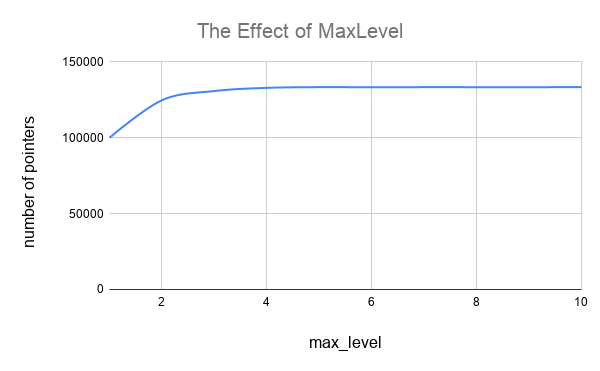Figure 14. The effect of MaxLevel on the number of pointers

### The Effect of p

Figure 15 shows the effect of p on the average search time. The suggested value for p is 0.25. As you can see increasing p does not help further reducing the search time.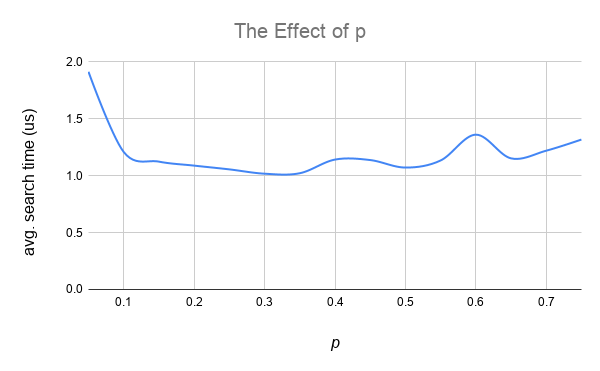Figure 15. The effect of p on average search time

Figure 16 shows the effect of p on the number of pointers. By increasing the p number of level increases, so more pointers will be needed.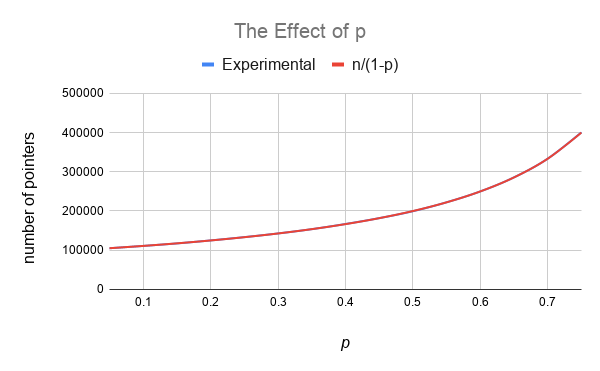Figure 16. The effect of p on the number of pointers

### The Effect of Locality of Reference and Finger Search

Figure 17 shows the effect of the locality of reference on the average search time. To enforce the locality of reference for our experiment, when we generate keys randomly for the search operation, we pick a key that is larger/smaller than the previous key by at most k.  From Figure 17 it is interesting to see that with just locality of reference even without using finger search, the search time significantly decreases. This improvement is due to the optimizations provided by the system such as caching. Figure 17 shows fingers slightly improve the search time.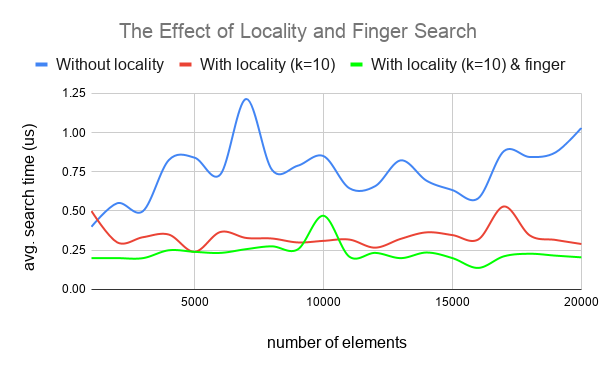Figure 17. The effect of the locality of reference and finger search

To further investigate the effect of fingers, Figure 18 compares the average search time with and without using fingers for different values of k. The average search time is smaller with fingers, and it increases as k increases.Figure 18. Skip list average search time with and without finger search

### Skip List vs. std::map

In this section, we compare the performance of our simple skip list with std::map that uses balanced trees to store sorted elements. Figure 19-21 shows the average time for insert, search, and erase, respectively. Finger optimization was disabled to obtain the following results. As you can see, our simple skip list implementation is performing even better than std::mapFigure 19. Skip list vs. std::map regarding the average insert timeFigure 20. Skip list vs. std::map regarding the average search time

Figure 21 is especially interesting, as it shows how rebalancing in std::map results in spikes in erasing time. On the other hand, skip list is less spiky and generally provides a shorter erase time.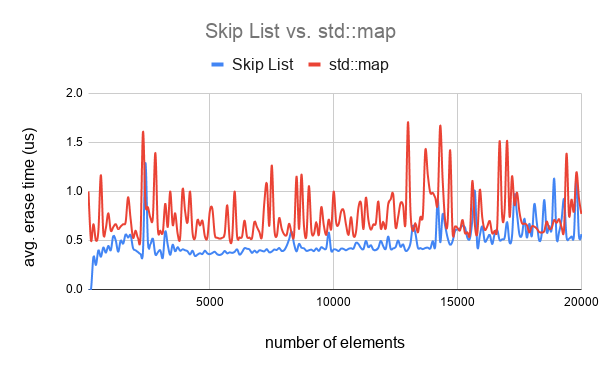Figure 21. Skip list vs. std::map regarding the average erase time

## Balanced search trees such as AVL-tree or Red-Black trees are alternatives to skip lists for storing sorted elements. Both skip lists and balanced trees provide logarithmic time complexity. Thus, the natural question is which one is better? Since both data structures provide the same asymptotic time complexity, there is no general agreement on which one is better, and we don't have a clear winner. Here also we don't need to call a winner, and we just provide some discussions from different perspectives. You can refer to more comprehensive comparisons in the literature.

### Simplicity

Simplicity can be a subjective matter, but most programmers (including myself) agree that skip list is significantly simpler than any balanced trees. As we saw above, a skip list is basically a simple linked list with some additional pointers that skip some elements in order. The search, insert, and erase algorithms are much simpler than the procedures that we need to perform to keep the balance of for the search trees.

Regarding simplicity, a very important aspect is to note that usually, non-recursive implementations of the balanced tree are significantly more complex than recursive versions. Thus, most programmers prefer to use recursive versions. However, recursive versions come with higher overhead, which results in higher constant factors. Thus, although the asymptotic time complexity remains the same, the constant factors of balanced trees ends up being higher than skip list. The constant factors are very important especially for sublinear complexities . Skip list algorithms are already iterative and simple. The fact that with a simple and not-so-efficient C++ implementation provided in  we are able to beat std::map, as shown in Figures 19-21, is very interesting.

### Space Efficiency

As explained above, the average number of pointers required for a skip list is 1/(1-p) per element. Thus, with p=0.5, we need 2n pointers, and with p=0.25 we need only 1.33n pointers. Note that our results above showing better performance of skip list compared with std::map obtained with p=0.25. For a balanced tree, each node needs at least 2 pointers, so we need 2n pointers.

### Concurrency

Although it is hard to call the winner between skip list and balanced trees, when it comes to concurrency we have a clear winner which is the skip list! In fact, the main motivation for inventing skip lists was the difficulty of designing effective concurrent trees . In this section, we review results provided by  on the concurrency of the skip list compared with the red-black tree. Figure 22 shows the performance of the skip list and red-black tree when we have low contention. Specifically, keys are selected from a very large keyspace of size 219. Each operation can be either search, insert, or delete with the following probabilities: search 75%, insert 12.5%, and delete 12.5%. They used different concurrency control techniques. In all cases, the skip list clearly outperforms the red-black tree.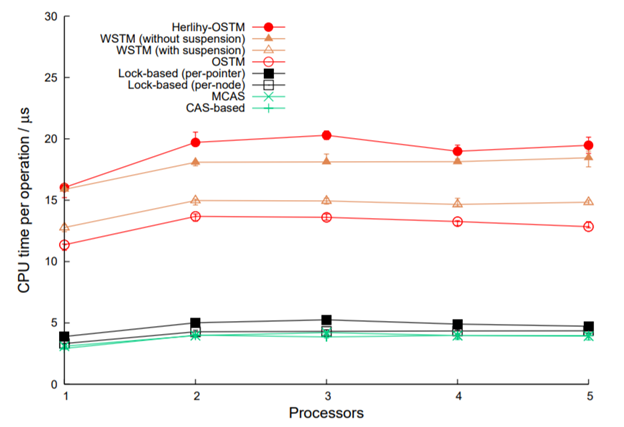(a) skip list

(b) red-black tree

 Figure 22. Performance of different concurrent versions of skip list vs. red-black tree with low contention 

Figure 23 shows the results for varying contention. Specifically, by increasing the mean set size the contention decreases, so CPU time decreases. Again, as it is clear, the skip list performs much better than the red-black tree.

Take away: If you want to store the sorted elements in a concurrent program, you should use a skip list.

If you are interested in learning more about skip list,  and  are very good sources. The implementation used in this blog post is for educational and experimental purposes. If you are interested to use the skip list in production, I suggest considering . To see a use case of skip lists, you can check the resolver cache in FoundationDB .

## Summary

Skip list is a powerful data structure that brings together the simplicity of linked list and the efficiency of the balanced search trees for storing sorted elements. We reviewed the skip list basic operations and saw how we can optimize the search operation by search fingers when we have locality of reference. With a simple C++ implementation, we were able to verify the theoretical results about the skip list. We also saw that this simple C++ implementation performs better than std::map for storing sorted elements.

## References

1. William Pugh, Skip Lists: A Probabilistic Alternative to Balanced Trees, Proceedings of the Workshop on Algorithms and Data Structures, Ottawa Canada, August 1989 (to appear in Comm. ACM).
2. William Pugh. A skip list cookbook. 1998.
4. Keir Fraser and Tim Harris. "Concurrent programming without locks." ACM Transactions on Computer Systems (TOCS) 25, no. 2 (2007): 5-es.
5. Jung-sang Ahn's GitHub, https://github.com/greensky00/skiplist
6. FoundationDB GitHub, https://github.com/apple/foundationdb/blob/master/fdbserver/SkipList.cpp